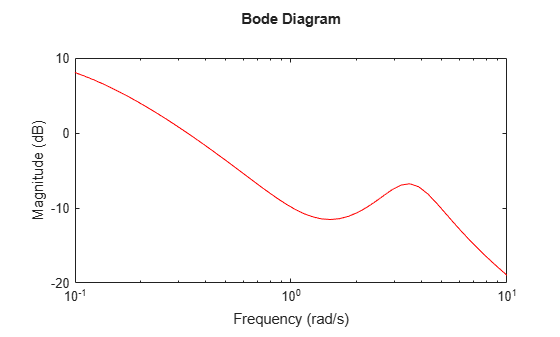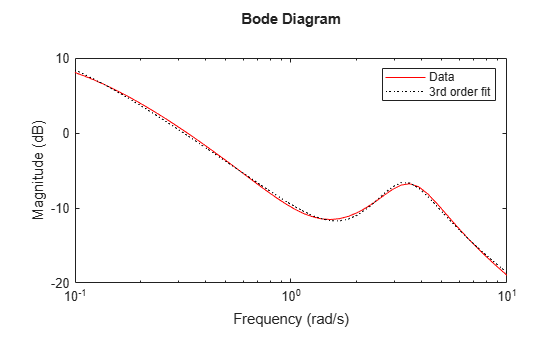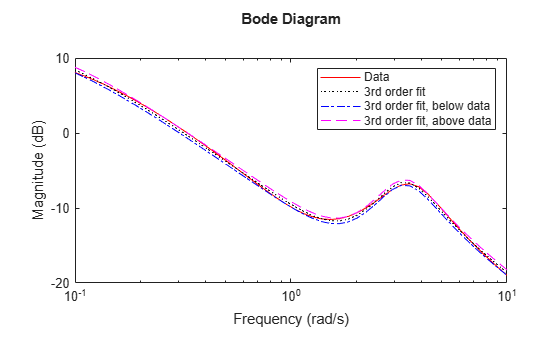# fitmagfrd

Fit frequency response magnitude data with minimum-phase state-space model using log-Chebyshev magnitude design

## Syntax

```B = fitmagfrd(A,N)
B = fitmagfrd(A,N,RD)
B = fitmagfrd(A,N,RD,WT)
B = fitmagfrd(A,N,RD,WT,C)
```

## Description

`B = fitmagfrd(A,N)` is a stable, minimum-phase `ss` object, with state-dimension `N`, whose frequency response magnitude closely matches the magnitude data in `A`. `A` is a 1-by-1 `frd` object, and `N` is a nonnegative integer.

`B = fitmagfrd(A,N,RD)` forces the relative degree of `B` to be `RD`. `RD` must be a nonnegative integer whose default value is `0`. You can specify the default value for `RD` by setting `RD` to an empty matrix.

`B = fitmagfrd(A,N,RD,WT)` uses the magnitude of `WT` to weight the optimization fit criteria. `WT` can be a `double`, `ss` or `frd`. If `WT` is a scalar, then it is used to weight all entries of the error criteria `(A-B)`. If `WT` is a vector, it must be the same size as `A`, and each individual entry of `WT` acts as a weighting function on the corresponding entry of (`A-B`). The default value for `WT` is 1, and you can specify it by setting `WT` to an empty matrix.

`B = fitmagfrd(A,N,RD,WT,C)` enforces additional magnitude constraints on `B`, specified by the values of `C.LowerBound` and `C.UpperBound`. These can be empty, `double` or `frd` (with `C.Frequency` equal to `A.Frequency`). If `C.LowerBound` is non-empty, then the magnitude of `B` is constrained to lie above `C.LowerBound`. No lower bound is enforced at frequencies where `C.LowerBound` is equal to -inf. Similarly, the `UpperBound` field can be used to specify an upper bound on the magnitude of `B`. If `C` is a `double` or `frd` (with `C.Frequency` equal to `A.Frequency`), then the upper and lower bound constraints on `B` are taken directly from `A` as:

• if C(w) == –1, then enforce abs(B(w)) <= abs(A(w))

• if C(w) == 1, then enforce abs(B(w)) >= abs(A(w))

• if C(w) == 0, then no additional constraint

where `w` denotes the frequency.

## Examples

collapse all

Create frequency response magnitude data from a fifth-order system.

```sys = tf([1 2 2],[1 2.5 1.5])*tf(1,[1 0.1]); sys = sys*tf([1 3.75 3.5],[1 2.5 13]); omega = logspace(-1,1); sysg = abs(frd(sys,omega)); bodemag(sysg,'r');```Fit the magnitude data with a minimum-phase, stable third-order system.

```ord = 3; b1 = fitmagfrd(sysg,ord); b1g = frd(b1,omega); bodemag(sysg,'r',b1g,'k:'); legend('Data','3rd order fit');```Fit the magnitude data with a third-order system constrained to lie below and above the given data.

```C2.UpperBound = sysg; C2.LowerBound = []; b2 = fitmagfrd(sysg,ord,[],[],C2); b2g = frd(b2,omega); C3.UpperBound = []; C3.LowerBound = sysg; b3 = fitmagfrd(sysg,ord,[],[],C3); b3g = frd(b3,omega); bodemag(sysg,'r',b1g,'k:',b2g,'b-.',b3g,'m--') legend('Data','3rd order fit','3rd order fit, below data',... '3rd order fit, above data')```Fit the magnitude data with a second-order system constrained to lie below and above the given data.

```ord = 2; C2.UpperBound = sysg; C2.LowerBound = []; b2 = fitmagfrd(sysg,ord,[],sysg,C2); b2g = frd(b2,omega); C3.UpperBound = []; C3.LowerBound = sysg; b3 = fitmagfrd(sysg,ord,[],sysg,C3); b3g = frd(b3,omega); bgp = fitfrd(genphase(sysg),ord); bgpg = frd(bgp,omega); bodemag(sysg,'r',b1g,'k:',b2g,'b-.',b3g,'m--',bgpg,'r--') legend('Data','3rd order fit','2d order fit, below data',... '2nd order fit, above data','bgpg')```## Limitations

This input `frd` object must be either a scalar 1-by-1 object or, a row, or column vector.

## Algorithms

`fitmagfrd` uses a version of log-Chebyshev magnitude design, solving

``` min f subject to (at every frequency point in A): |d|^2 /(1+ f/WT) < |n|^2/A^2 < |d|^2*(1 + f/WT) ```

plus additional constraints imposed with `C`. `n, d` denote the numerator and denominator, respectively, and `B = n/d`. `n` and `d` have orders (`N-RD`) and `N`, respectively. The problem is solved using linear programming for fixed `f and` bisection to minimize `f`. An alternate approximate method, which cannot enforce the constraints defined by `C`, is ```B = fitfrd(genphase(A),N,RD,WT)```.

## References

Oppenheim, A.V., and R.W. Schaffer, Digital Signal Processing, Prentice Hall, New Jersey, 1975, p. 513.

Boyd, S. and Vandenberghe, L., Convex Optimization, Cambridge University Press, 2004.